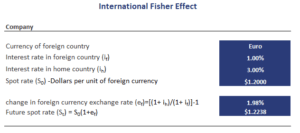# International Fisher Effect

The International Fisher Effect (IFE), sometimes also called the Fisher-open effect, is an important hypothesis in finance. The hypothesis was first proposed by the famous economist Irving Fisher in the 1930s. This is the same economist who also proposed the quantity theory of money and the Fisher index. These theories argue that price level in an economy is directly and proportionally related to the money supply and the level of inflation, respectively.

The International Fisher effect uses the insights from the quantity theory of money and the Fisher effect to make predictions on how exchange rates between open economies with no fixed exchange rates (i.e. floating exchange rates) can be expected to change. On this page, we explain the international Fisher effect and its relationship with interest rate parity. We also provide the formula and finally an international Fisher effect example to give some intuition on how to apply the formula. The calculation can easily be done using Excel, which we illustrate in the Excel file at the bottom of this page.

## International Fisher effect definition

What is the international fisher effect? The theory states that

differences in nominal interest rates should reflect expected changes in the spot exchange rate between different countries.

Thus, the currency rate of the country with the higher interest rate is expected to depreciate relative to the currency of the country with the lower nominal interest rate. This is because nominal interest rates include a component that compensates investors for expected inflation.

Higher inflation means that the purchasing power of the currency will decrease. As a consequence, and assuming the real interest rates are the same in both countries, the currency with the highest inflation rate should drop in value compared to the currency with the lower inflation rate.

## Equation

The equation is the following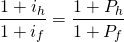where ih is het nominal interest rate of the home country, if is the interest rate of the foreign country, Ph is het rate of inflation of the home country, Pf is the rate of inflation of the foreign country.

Thus, the expected change in the inflation rate equals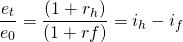where e is the spot exchange rate in currency units of foreign per unit of home currency.

## International Fisher Effect example

As an example, consider a country with with a nominal interest rate of 12% (ih) and a country with a nominal interest rate of 9% (if). The current spot exchange rate is f = 1.5Q

Then the expected exchange rate equals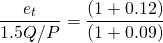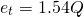## Fisher Effect calculator

Using Excel, it is extremely simple to build a calculator that calculates the expected change rate change given nominal interest rates in both countries. Below we illustrate the necessary calculations